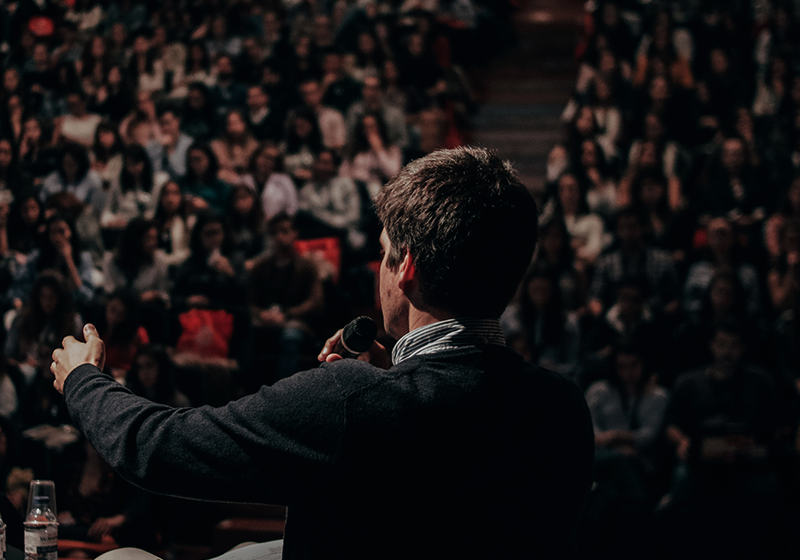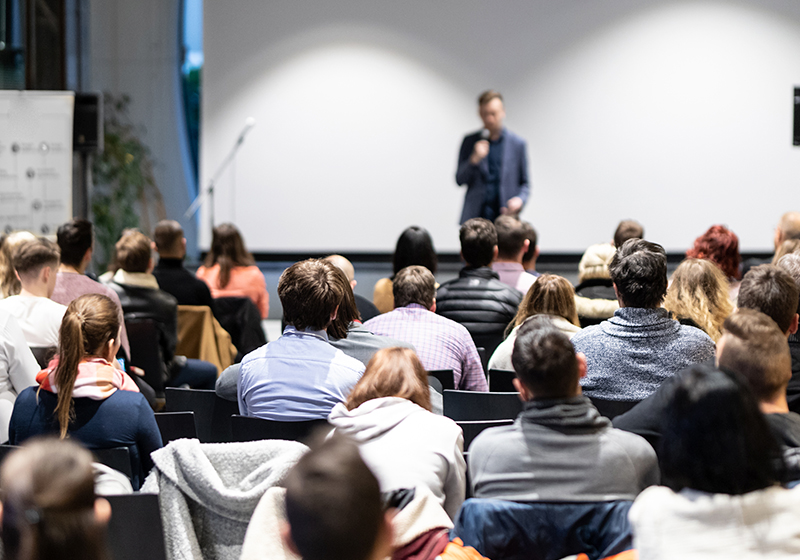Título: "Lax equivalence principle for problems in fluid dynamics!"
Data: 22/11/2023
Horário: 12:00h
Sala: C-116

Palestrante: Eduard Feireisl (Institute of Mathematics of the Czech Academy of Sciences)

Resumo: We discuss possible generalizations of the celebrated Lax equivalence principle: stability consistency yields convergence'' to a class of nonlinear problems arising in fluid mechanics. In particular, we establish convergence of approximate schemes for problems with uncertain data.Titulo: "Ingham type theorems and observability problems"
Palestrante: Vilmos Komornik (University of Strasbourg)
Data: 13/09/2023
Horário: 12h
Sala: C-119

Resumo: We report on some joint works with Claudio Baiocchi and Paola Loreti on the application of non-harmonic Fourier series to control theory.Título: On the optimal control of non-Newtonian fluids.

Data: 12/07/2023
Horário: 12:00h
Local: C-116
Palestrante: Fernanda Cipriano (Universidade Nova de Lisboa)

Resumo:

We discuss the optimal control problems of flows governed by the incompressible deterministic and stochastic third grade fluid equations with Navier-slip boundary conditions.

After recalling the results on the well-posedness of the state equations, we study the existence and the uniqueness of solution to the linearized state and adjoint equations. Furthermore, we present a stability result for the state, and show that the solution of the linearized equation coincides with the Gâteaux derivative of the control-to-state mapping. Next, we prove the existence of an optimal solution and establish the first order optimality conditions.

In the deterministic case, an uniqueness result of the coupled system constituted by the state equation, the adjoint equation and the first order optimality condition is established, under sufficiently large intensity of the cost.

This is a joint work with Y. Tahraoui.

Bibliography:

 A. Almeida, N. Chemetov and F. Cipriano, Uniqueness for optimal control problems of two-dimensional second grade fluids. EJDE, Vol. 2022, No. 22, 1-12 (2022)

 N. Chemetov, F. Cipriano, Optimal control for two-dimensional stochastic second grade fluids. Stochastic Processes Appl., 128, Issue 8, 2710-2749 (2018)

 F. Cipriano, P. Didier, S. Guerra, Well-posedness of stochastic third grade fluid equation. J. Diff. Eq., 285, 496-535 (2021)

 Y. Tahraoui and F. Cipriano, Optimal control of two dimensional third grade fluids. J. Math. Anal. Appl., 523, 127032, (2023)

 Y. Tahraoui and F. Cipriano, Local strong solutions to the stochastic third grade fluid equations with Navier Boundary conditions,
https://doi.org/10.48550/arXiv.2302.05672Título: Spectrum of differential operators with elliptic adjoint on a scale of localized Sobolev spaces.

Data: 14/06/2023
Horário: 12:00h
Local: C-116
Palestrante: Luís Salge (UFRJ)

Resumo: In this work we present a complete study about the spectrum of a constant coefficients differential operator of order m a positive integer, a(D), whose adjoint a(D)* is elliptic, seen as a pseudo-differential operator on an interval I.  The best conclusions we obtain are when we consider the Laplace operator on an interval I defined on a Sobolev space of order 2, i.e., H^{2}_{0}(I) with the topology induced by the L^{2}_{loc} which is a Fréchet space. For it, we calculate its closure and their types of spectrum.

Titulo: Propriedades matemáticas do fluxo de espuma em meios porosos.Palestrante: Luis Fernando Lozano G. (LAMAP - UFJF)
Data: 09/08/2023
Horário: 12h
Sala: C-116

Resumo: AQUI